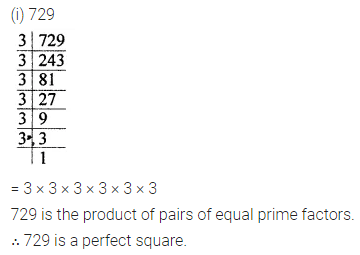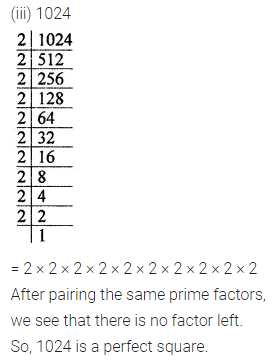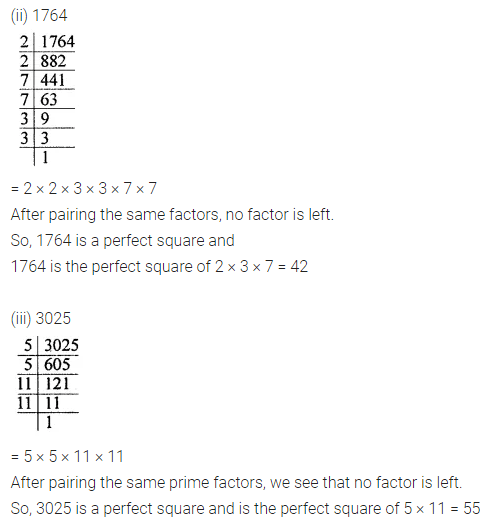# ML Aggarwal Class 8 Solutions for ICSE Maths Chapter 3 Squares and Square Roots Ex 3.1

## ML Aggarwal Class 8 Solutions for ICSE Maths Chapter 3 Squares and Square Roots Ex 3.1

Question 1.
Which of the following natural numbers are perfect squares? Give reasons in support of your answer.
(i) 729
(ii) 5488
(iii) 1024
(iv) 243
Solution:Question 2.
Show that each of the following numbers is a perfect square. Also, find the number whose square is the given number.
(i) 1296
(ii) 1764
(iii) 3025
(iv) 3969
Solution:Question 3.
Find the smallest natural number by which 1008 should be multiplied to make it a perfect square.
Solution:Question 4.
Find the smallest natural number by which 5808 should be divided to make it a perfect square. Also, find the number whose square is the resulting number.
Solution: